﻿ 20-sim webhelp > Library > Iconic Diagrams > Mechanical > Rotation > Gears > UniversalCoupling

# UniversalCoupling

## Library

Iconic Diagrams\Mechanical\Rotation\Gears

## Use

Domains: Continuous. Size: 1-D. Kind: Iconic Diagrams (Rotation).

## Description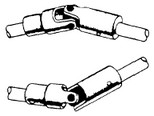Universal Joint couplings are used to couple axes which are not aligned. The can be used in single or double set-up. Consider the single Universal Joint coupling, shown in the picture below.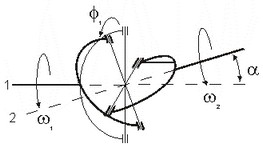Due to the nature of the coupling, the output angular velocity w1 will show a sinusoidal ripple, compared to the input angular velocity w2: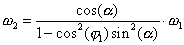with α the angle between the two axes and j1 the initial rotation of the input axis. The initial rotation j1 is defined with respect to a plane that is spanned by the axes (1 and 2).

When two Universal Joint couplings are combined, the angular velocity of the output axis will be equal to the angular velocity of the input axis (e.g. no ripple) when two conditions are met. When the output axis of the first coupling is connected to the input axis of the second coupling, these conditions are:

 1 For both couplings the absolute value of the angle α should be equal.
 2 The difference in initial rotation of both couplings ( j1 - j2) should be equal to +p/2 [rad] or -p/2 [rad].

Two alignments are shown in the figure below.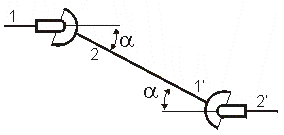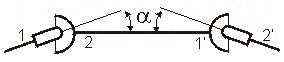α = 0.35, α' = -0.35 , j1 = 0, j1' = p/2 [rad] α = 0.35, α' = 0.35 , j1 = 0, j1' = p/2 [rad]

## Interface

 Ports Description p1, p2 The input and output axes (Rotation) Causality Input p1 not equal p2 Parameters alpha The angle between the two axes (α). Initial Values phi1_initial The initial rotation of the input axis (j1).